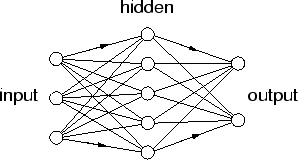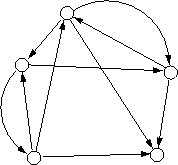Next: 1.5.2 Internal models Up: 1.5 Learning of sensorimotor Previous: 1.5 Learning of sensorimotor

## 1.5.1 Neural networks

Artificial neural networks are simplified models of neurons and their connections in the brain. This section just provides a classification of different structures and functions. The basic mathematics and applications can be found, for example, in Hertz et al. (1991) and Haykin (1998).

The structure of neural networks can be divided into two classes: feed-forward networks and recurrent neural networks (figure 1.1). The first have connections in one direction from input to output. Here, the prominent type is the multi-layer perceptron (MLP). It has an input layer, one hidden layer or many hidden layers, and an output layer. MLPs approximate functions. Therefore, they map from one input pattern to just one output pattern (one-to-one or many-to-one mappings), but they fail on one-to-many mappings.

Figure 1.1: Two basic neural-network structures: feed-forward (left) and recurrent (right).In contrast, recurrent neural networks have feed-back connections. Therefore, they do not approximate functions, instead their state (the values of all neurons) changes in time1.7. The convergence of the state depends on the connections. Partially recurrent networks that feed the network output back into the input oscillate. They have been used for time-series prediction. A prominent example is the Elman network (Elman, 1990). This network has an MLP structure with an additional context layer, which receives delayed input from the hidden layer and therefore acts like a memory (see also figure 1.5). Fully interconnected networks with symmetric weights converge to a stable state. These networks have been used for pattern completion (Hopfield, 1982). Patterns can be stored as stable states.

Neural networks learn either in a supervised way with a teacher, or unsupervised. In supervised learning, a teacher provides a target value (at the output) for every input value. Examples are error backpropagation for feed-forward networks and backpropagation-through-time for recurrent networks (Hertz et al., 1991). In contrast, unsupervised learning methods do not need target values. They either store training patterns (Hopfield network), or try to find structure in the training set. Structure can be found by assuming that the training patterns lie on a lower-dimensional manifold embedded in the pattern space. For example, an auto-associative network--an MLP whose input and output are identical--constrains the output to a manifold whose local dimensionality equals the number of neurons in the smallest hidden layer (Hertz et al., 1991; Kambhatla and Leen, 1997). Further, the self-organizing-map algorithm (Kohonen, 1995) fits a grid to the embedded manifold (see section 1.5.5). Finally, networks that do a `principal component analysis' (Diamantaras and Kung, 1996) exploit that the variance of the patterns is restricted to a few principal directions (see section 2.1).Next: 1.5.2 Internal models Up: 1.5 Learning of sensorimotor Previous: 1.5 Learning of sensorimotor
Heiko Hoffmann
2005-03-22